Register for Maths, Science, English, Reasoning Olympiad Exams Register here | Book Free Demo for Live Olympiad Classes here | Check Olympiad Exam Dates here | Buy Practice Papers for IMO, IOM, HEO, IOEL etc here | Login here to participate in all India free mock test on every Saturday

# Problems Based on Figures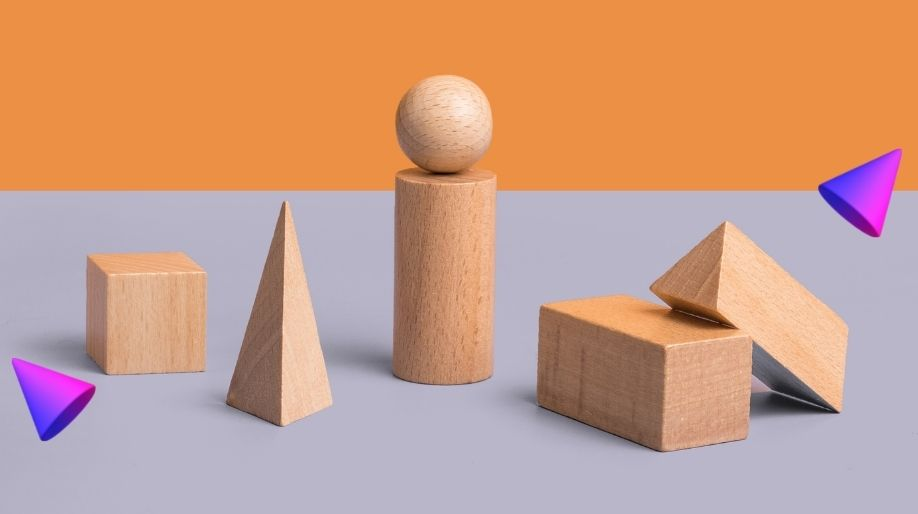There are a variety of shapes present in an object. An object mostly has one or more geometrical shapes like the following.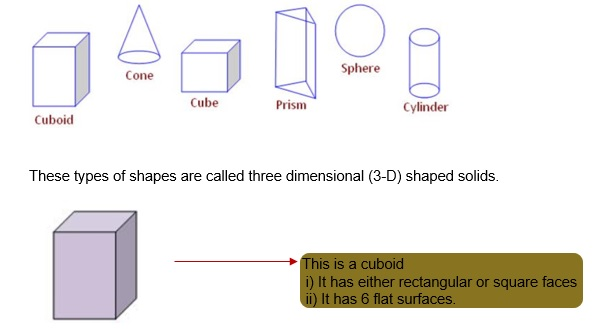These types of shapes are called three dimensional (3-D) shaped solids.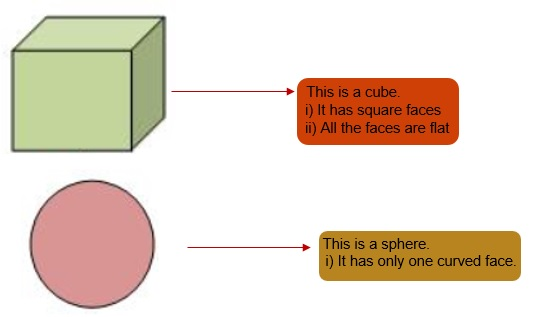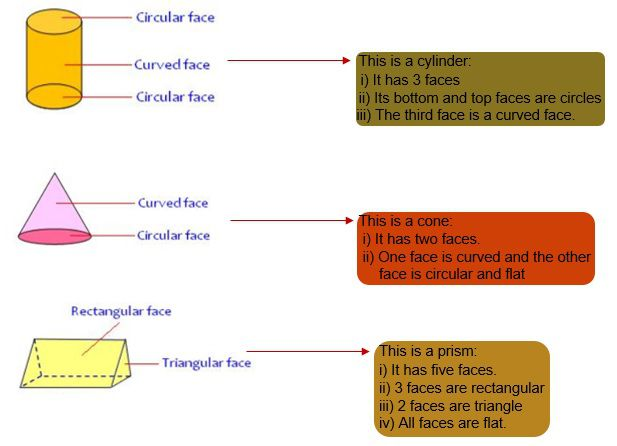# Practice these questions

Q1) Colour all the cones RED, all the spheres YELLOW, all the cylinders BLUE and all the cubes GREEN.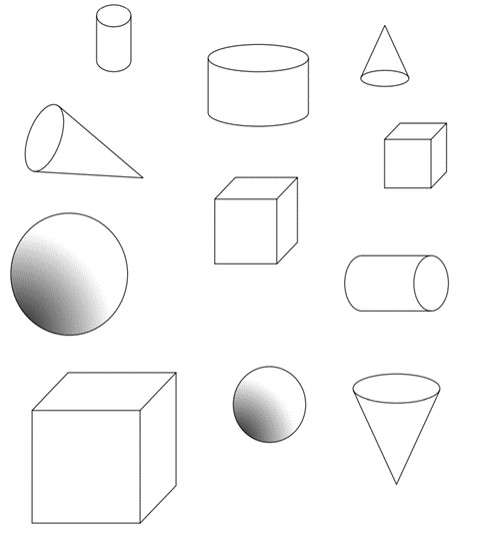Q2) Circle the shape which best matches the real-life object in the picture.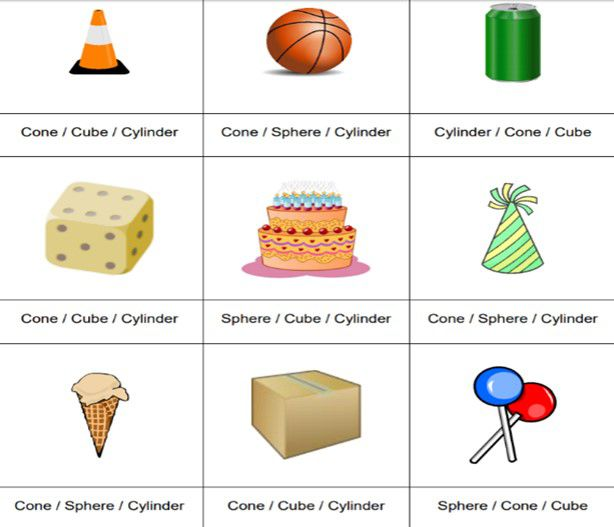Q3) Fill in the following table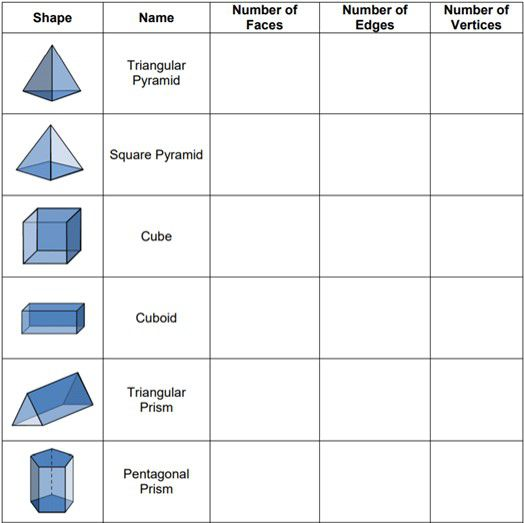Q4) Circle the shape with more faces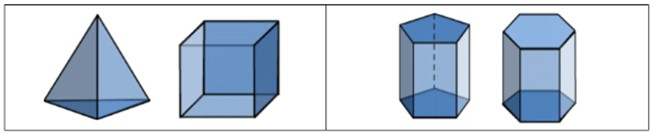## Quiz for Problems Based on Figures

 Q.1 A sphere is ____ dimension shape.a) three b) two c) one d) four
 Q.2 An ice cube resemble which geometric shape?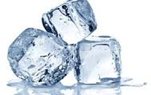a) sphere b) circle c) cube d) pyramid
 Q.3 A marker is an example of which geometric shape?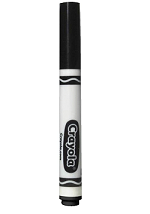a) cone b) square c) cylinder d) sphere
 Q.4 A cardboard box is an example of which geometric shape?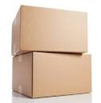a) circle b) hemisphere c) sphere d) cuboid
 Q.5 A pencil tip is an example of which geometric shape?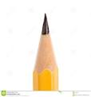a) cube b) pyramid c) cone d) cylinder
 Q.6 Which among the following is an example of a cone? a) a mobile phone b) box c) birthday cap d) ice cube
 Q.7 A cube is made up of which 2-dimensional geometric shape? a) polygon b) circle c) square d) rectangle
 Q.8 A rectangular prism is also known as- a) cuboid b) cube c) pyramid d) sphere
 Q.9 We can stack___ dimensional shape figure one upon the another. a) three b) four c) two d) one
 Q.10 Circles do not have- a) radius b) angles c) area d) circumference